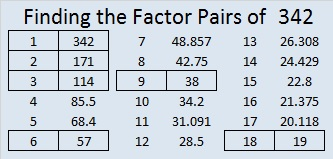# 342 and Level 6

• 342 is a composite number.
• Prime factorization: 342 = 2 x 3 x 3 x 19, which can be written 342 = 2 x (3^2) x 19
• The exponents in the prime factorization are 1, 2, and 1. Adding one to each and multiplying we get (1 + 1)(2 + 1)(1 + 1) = 2 x 3 x 2 = 12. Therefore 342 has exactly 12 factors.
• Factors of 342: 1, 2, 3, 6, 9, 18, 19, 38, 57, 114, 171, 342
• Factor pairs: 342 = 1 x 342, 2 x 171, 3 x 114, 6 x 57, 9 x 38, or 18 x 19
• Taking the factor pair with the largest square number factor, we get √342 = (√9)(√38) = 3√38 ≈ 18.493342 = 18 × 19, which means it is the sum of the first 18 even numbers.

• Thus, 2 + 4 + 6 + 8 + . . .  + 32 + 34 + 36 = 342

It also means that we are halfway between 18² and 19², or halfway between 324 and 361. The average of those two numbers is 342.5.

AND it means that 18² + 19² – 1 = 2(342) = 2(18 × 19)

Here are a few things to think about before you try to solve the puzzle I’ve posted below:

• Will you decide to use the 6 or the 9 as the common factor of 72 and 54?
• Will 5 or 10 be the common factor of 20 and 50?
• The common factor for 27 and 9 could be 9 or it could be 3,
• and the common factor for 32 and 24 is either 4 or 8.
• Can you use logic to come up with the correct factor choices to make this puzzle work as a multiplication table?Print the puzzles or type the factors on this excel file: 12 Factors 2014-12-29This site uses Akismet to reduce spam. Learn how your comment data is processed.